# Krull intersection theorem for modules

This article defines a result where the base ring (or one or more of the rings involved) is Noetherian
View more results involving Noetherianness or Read a survey article on applying Noetherianness
This fact is an application of the following pivotal fact/result/idea: Artin-Rees lemma
View other applications of Artin-Rees lemma OR Read a survey article on applying Artin-Rees lemma
This fact is an application of the following pivotal fact/result/idea: Cayley-Hamilton theorem
View other applications of Cayley-Hamilton theorem OR Read a survey article on applying Cayley-Hamilton theorem

## Statement

Let$R$ be a Noetherian ring and$I$ be an ideal inside$R$. Suppose$M$ is a finitely generated module over$R$. Then, we have the following:

1. Let$N = \bigcap_{j=1}^\infty I^j M$. Then,$IN = N$
2. There exists$r \in I$ such that$(1 - r)N = 0$

## Proof

### The intersection equals its product with$I$

We first show that the intersection equals its product with$I$. This is the step where we se the Artin-Rees lemma.

Let: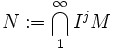$N := \bigcap_1^\infty I^jM$

Now consider the filtration: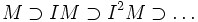$M \supset IM \supset I^2M \supset \ldots$

this is an$I$-adic filtration and the underlying ring is Noetherian, hence by the Artin-Rees lemma, the following filtration is also$I$-adic: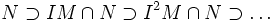$N \supset IM \cap N \supset I^2M \cap N\supset \ldots$

Since each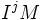$I^jM$ contains$N$, the filtration below is the same as the filtration: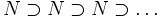$N \supset N \supset N \supset \ldots$

This being$I$-adic forces that$IN = N$.

### Finding the element$r$

Since$IN = N$, we can find an element$r \in I$ such that$(1 - r)N = 0$. This is an application of the Cayley-Hamilton theorem: we first find the Cayley-Hamilton polynomial, then observe that$1$ is a root of the polynomial, and then take the negative of the sum of all coefficients of higher degree terms.